The K5 Learning Blog urges parents to be pro-active in helping their children reach their full academic potential.

K5 Learning
provides free worksheets, workbooks and an online reading and math program for kindergarten to grade 5 students.# How to Solve Division Word Problems

In grade 3 students move onto more complex word problems using multiplication and division. Below we’ll take you through a step-by-step guide on how to solve a simple division word problem.

## Turn the English into Algebra

The first rule of any word problem is to think in math terms. To do that, you’ll need to:

• Read the whole word problem.
• Write down what is asked for. (As students work through the word problem, they can get lost and start to work in a different direction. Having the actual request written down, will help to keep them focus on the goal.)
• Sketch out the word problem, if possible.
• the signs where you see key words. For example, write + where you see add, increase, combine, or – where you see less, difference, reduce.
• Find or work out any formulas.

## Division word problem example

Let’s work through a division word problem:

Katie has 700 candies. She gave away 175 candies to her classmates. She put the remaining candies in five separate bags. How may candies are there in each bag?

First, read through the whole word problem once more.

Now, what is being asked? We want to know how many candies are in each of the 5 bags.

Let’s sketch it out: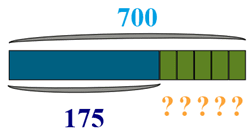Write down the math signs where you see key words in the text: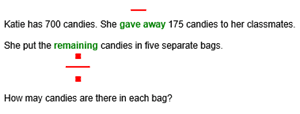In the above, we can see there are two calculations to be completed.

First, we need to find out how many candies there are left after Katie hands out 175 candies to her classmates: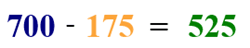Now we know there are 525 candies remaining. Next, we need to work out how many candies she put in each of the 5 bags: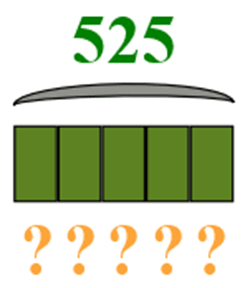We need to divide the 525 candies by 5 bags: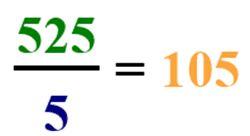The answer is there are 105 candies in each bag.

For practice, we have division word problems worksheets in our grade 3 word problems section.